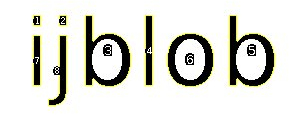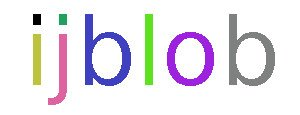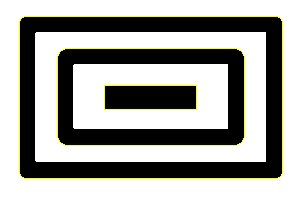# IJ BlobThe content of this page has not been vetted since shifting away from MediaWiki. If you’d like to help, check out the how to help guide!File ij-blob.jar [1 ] Source Github [2 ]

## Purpose

The IJBlob library indentifying connected components in binary images. The algorithm used for connected component labeling is:

Chang, F. (2004). A linear-time component-labeling algorithm using contour tracing technique. Computer Vision and Image Understanding, 93(2), 206–220. <doi:10.1016/j.cviu.2003.09.002>

A connected component is a set of pixels which are connected by its 8-neigherhood and is often called a “blob”. An Example:The image above contains 8 marked blobs. Also the holes (and the contours of the holes) of the two Bs and the O are identified. It is also possible to get a color labeled image:In addition ijblob identifies nested objects:If you are using IJBlob in a scientific publication, please cite:

Wagner, T and Lipinski, H 2013. IJBlob: An ImageJ Library for Connected Component Analysis and Shape Analysis. Journal of Open Research Software 1(1):e6, DOI: http://dx.doi.org/10.5334/jors.ae

The Shape Filter plugin uses the ij-blob library to characterize and filter objects in binary scenes by its shape. Therefore, several features are calculated as shown below.

## Features of IJBlob

• Filter Framework
• Extract the outer contour of each blob.
• Extracts also all inner contours of each blob (holes).
• Detects nested objects (blob in blob).
• Many shape features

## Shape Features

• Area ($$A$$): The area enclosed by the outer contour of an object.
• Area Convex Hull ($$C$$): The area enclosed by the convex hull of the outer contour of an object.
• Perimeter ($$P$$): The perimeter of the outer contour of an object.
• Perimeter Convex Hull ($$H$$): The perimeter of the convex hull of the particle.
• Feret Diameter: The maximum distance between the two parallel tangents touching the particle outline in all directions.
• Min. Feret Diameter: the minimum distance between the two parallel tangents touching the particle outline in all directions.
• Max. Inscr. Circle Diameter: The diameter of the maximum inscribed circle.
• Long Side Minimum Bounding Rectangle: The larger side of the minimum bounding rectangle.
• Short Side Minimum Bounding Rectangle: The smaller side of the minimum bounding rectangle.
• Aspect Ratio: Defined as $$L/S$$
• Area to Perimeter Ratio: Defined as $$A/P$$
• Circularity: Defined as $$P^{2}/A$$
• Elongation: Defined as $$1 - S/L$$
• Convexity: Defined as $$H/P$$
• Solidity: Defined as $$A/C$$
• Number of Holes: The number of holes inside an object.
• Thinnes Ratio: Inverse proportional to the circularity. Furthermore it normed. It is defined as $$4\pi A/P^{2}$$
• Contour Temperatur: It has a strong relationship to the fractal dimension, defined as $$\left(log_{2}\left(\frac{2P}{P-H}\right)\right)^{-1}$$
• Orientation: The orientation of the major axis from in grad (measured counter clockwise from the positive x axis).
• Fractal Box Dimension: Estimated fractal dimension by the box count algorithm. The default box-sizes are “2,3,4,6,8,12,16,32,64”.

# Examples

## Example 1: Extract the connected components of an image and read the perimeter of a blob

import ij.blob.*;
...
private ManyBlobs allBlobs;
...
public void someMethod(ImagePlus imp) {
ManyBlobs allBlobs = new ManyBlobs(imp); // Extended ArrayList
allBlobs.setBackground(0); // 0 for black, 1 for 255
allBlobs.findConnectedComponents(); // Start the Connected Component Algorithm
allBlobs.get(0).getPerimeter(); // Read the perimeter of a Blob
}


## Example 2: Example 2: Filter blobs by blob features

In IJBlob 1.1 a filter framework was introduced. Each build-in blob feature has a static identifier (in this example “GETENCLOSEDAREA”) which contains the method name.

import ij.blob.*;
...
private ManyBlobs allBlobs;
...
public void someMethod(ImagePlus imp) {
/* Extended ArrayList */
ManyBlobs allBlobs = new ManyBlobs(imp);

/* Start the Connected Component Algorithm */
allBlobs.findConnectedComponents();

/* Return all blobs with an area between 20 and 100 pixel² */
ManyBlobs filteredBlobs = allBlobs.filterBlobs(20,100,
Blob.GETENCLOSEDAREA);
}


IJBlob 1.1 is easily expandable by your own features. First you have to derive a feature class from the “CustomBlobFeature” class. The feature class can also contain multiple features. With the “getBlob()” method you get the reference to the Blob and have full access to the contour data and the other features. Please note: If your feature method have primitive data types (int/float/double) as parameters you have to use the wrapper classes (Integer/Float/Double).

import ij.blob.*;
public class ExampleBlobFeature extends CustomBlobFeature {
public double myFancyFeature(Integer a, Float b){
double feature = b*getBlob().getEnclosedArea()*a;
return feature;
}
public int mySecondFancyFeature(Integer a, Double b){
int feature = (int)(b*getBlob().getAreaToPerimeterRatio() *a);
return feature;
}
}


## Example 4: Add detected blobs to the ROI manager

Find all blobs:

ManyBlobs allBlobs = new ManyBlobs(imp);
allBlobs.findConnectedComponents();


Open the ROI-Manager:

Frame frame = WindowManager.getFrame("ROI Manager");
if (frame == null)
IJ.run("ROI Manager...");
frame = WindowManager.getFrame("ROI Manager");
RoiManager roiManager = (RoiManager) frame;


Convert the outer contour to a ROI and add it to the ROI manager:

for (int i = 0; i < allBlobs.size(); i++) {
Polygon p = allBlobs.get(i).getOuterContour();
int n = p.npoints;
float[] x = new float[p.npoints];
float[] y = new float[p.npoints];
for (int j=0; j<n; j++) {
x[j] = p.xpoints[j]+0.5f;
y[j] = p.ypoints[j]+0.5f;
}
Roi roi = new PolygonRoi(x,y,n,Roi.TRACED_ROI);
Roi.setColor(Color.green);
}


# Who used this plugin?

This is a list of publication who used ijblob / shape filter plugin:

Kulbacka, J., Kulbacki, M., Segen, J., Chodaczek, G., Dubinska-Magiera, M., & Saczko, J. (2016). Cellular Nuclei Differentiation Evaluated by Automated Analysis of CLSM Images. Intelligent Information and Database Systems Lecture Notes in Computer Science, 407-416. <doi:10.1007/978-3-662-49390-8_40>

Ullrich, M., Haša, J., Hanuš, J., Šoóš, M., & Štěpánek, F. (2016). Formation of multi-compartmental particles by controlled aggregation of liposomes. Powder Technology, 295, 115-121. <doi:10.1016/j.powtec.2016.03.021>

Kuleesha, Y., Puah, W. C., Lin, F., & Wasser, M. (2014). FMAj: A tool for high content analysis of muscle dynamics in Drosophila metamorphosis. BMC Bioinformatics, 15(Suppl 16). <doi:10.1186/1471-2105-15-s16-s6>

Cinquin, B., Maigre, L., Pinet, E., Chevalier, J., Stavenger, R. A., Mills, S., . . . Pagès, J. (2015). Microspectrometric insights on the uptake of antibiotics at the single bacterial cell level. Sci. Rep. Scientific Reports, 5, 17968. <doi:10.1038/srep17968>

Starborg, T., Kalson, N. S., Lu, Y., Mironov, A., Cootes, T. F., Holmes, D. F., & Kadler, K. E. (2013). Using transmission electron microscopy and 3View to determine collagen fibril size and three-dimensional organization. Nat Protoc Nature Protocols, 8(7), 1433-1448. <doi:10.1038/nprot.2013.086>